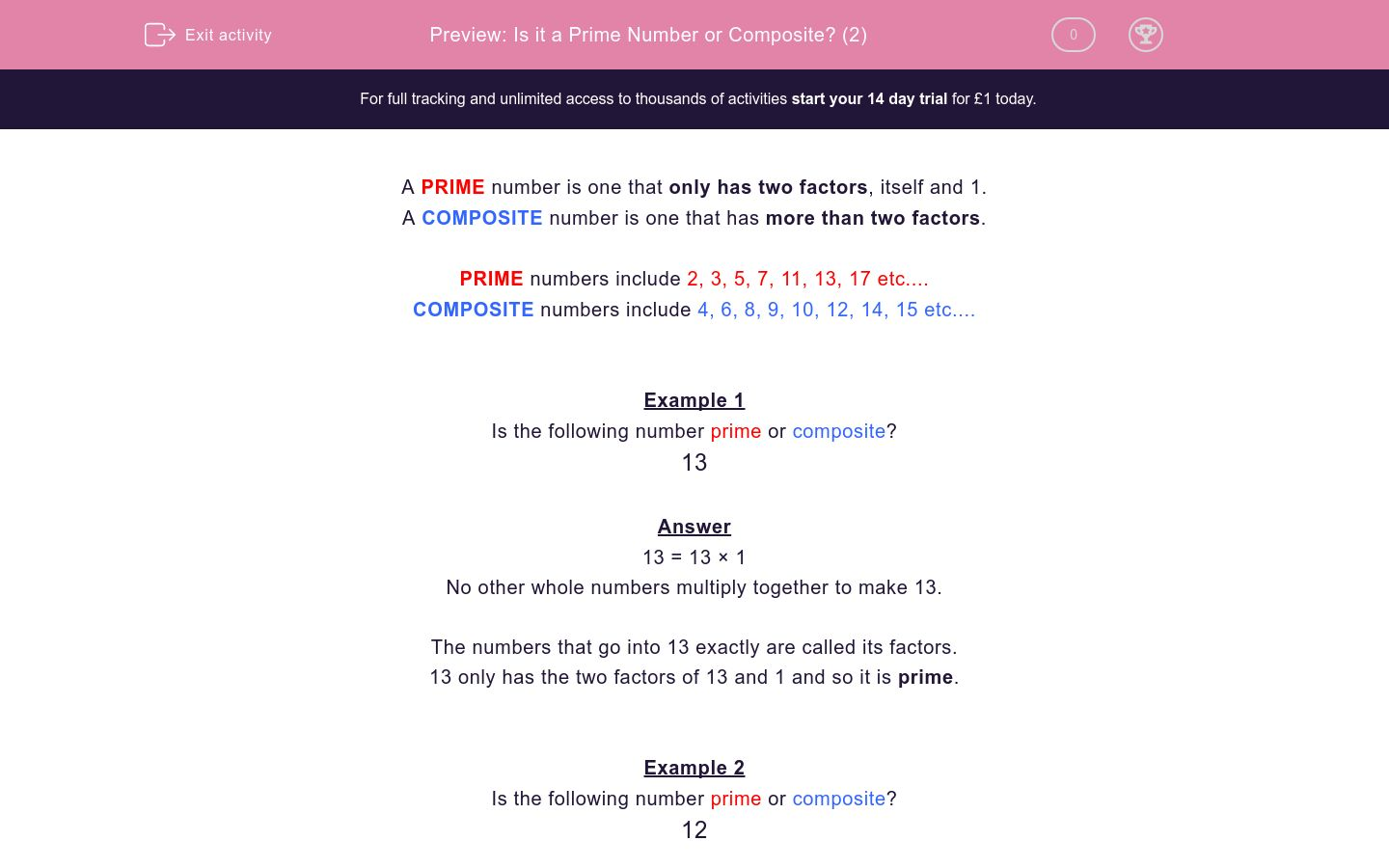# Is it a Prime Number or Composite? (2)

In this worksheet, students decide whether or not a given number is prime or composite.Key stage:  KS 2

Curriculum topic:   Maths and Numerical Reasoning

Curriculum subtopic:   Special Numbers

Difficulty level:### QUESTION 1 of 10

A PRIME number is one that only has two factors, itself and 1.

A COMPOSITE number is one that has more than two factors.

PRIME numbers include 2, 3, 5, 7, 11, 13, 17 etc....

COMPOSITE numbers include 4, 6, 8, 9, 10, 12, 14, 15 etc....

Example 1

Is the following number prime or composite?

13

13 = 13 × 1

No other whole numbers multiply together to make 13.

The numbers that go into 13 exactly are called its factors.

13 only has the two factors of 13 and 1 and so it is prime.

Example 2

Is the following number prime or composite?

12

12 = 12 × 1

12 = 6 × 2

12 = 4 × 3

The numbers that go into 12 exactly are called its factors.

12 has factors of 12, 6, 4, 3, 2 and 1 and so it is composite.

Is the following number prime or composite?

13

composite

prime

Is the following number prime or composite?

17

composite

prime

Is the following number prime or composite?

19

prime

composite

Is the following number prime or composite?

14

composite

prime

Is the following number prime or composite?

23

composite

prime

Is the following number prime or composite?

15

composite

prime

Is the following number prime or composite?

16

composite

prime

Is the following number prime or composite?

18

composite

prime

Is the following number prime or composite?

29

composite

prime

Is the following number prime or composite?

25

prime

composite

• Question 1

Is the following number prime or composite?

13

prime
EDDIE SAYS
13 only has factors of 13 and 1 and so is prime.
• Question 2

Is the following number prime or composite?

17

prime
EDDIE SAYS
17 only has factors of 17 and 1 and so is prime.
• Question 3

Is the following number prime or composite?

19

prime
EDDIE SAYS
19 only has factors of 19 and 1 and so is prime.
• Question 4

Is the following number prime or composite?

14

composite
EDDIE SAYS
14 has factors of 14, 7, 2 and 1 and so is composite.
• Question 5

Is the following number prime or composite?

23

prime
EDDIE SAYS
23 only has factors of 23 and 1 and so is prime.
• Question 6

Is the following number prime or composite?

15

composite
EDDIE SAYS
15 has factors of 15, 5, 3 and 1 and so is composite.
• Question 7

Is the following number prime or composite?

16

composite
EDDIE SAYS
16 has factors of 16, 8, 4, 2 and 1 and so is composite.
• Question 8

Is the following number prime or composite?

18

composite
EDDIE SAYS
18 has factors of 18, 9, 6, 3, 2 and 1 and so is composite.
• Question 9

Is the following number prime or composite?

29

prime
EDDIE SAYS
29 only has factors of 29 and 1 and so is prime.
• Question 10

Is the following number prime or composite?

25

composite
EDDIE SAYS
25 has factors of 25, 5 and 1 and so is composite.
---- OR ----

Sign up for a £1 trial so you can track and measure your child's progress on this activity.

### What is EdPlace?

We're your National Curriculum aligned online education content provider helping each child succeed in English, maths and science from year 1 to GCSE. With an EdPlace account you’ll be able to track and measure progress, helping each child achieve their best. We build confidence and attainment by personalising each child’s learning at a level that suits them.

Get started